# Introduction

The user guide highlighted the basic use of the prevalence simulation methodology, demonstrating that the prevalence process is a result of two distinct processes: incidence and survival. rprev allows for custom specification of both of these systems, in addition to providing default implementations that work well in many situations. This vignette acts as a guide to assessing the modelling assumptions made when using these default models, including the use of diagnostic functions provided with rprev.

First, we will inspect the consistency of incidence data across the range of the registry and determine if it can be appropriately modelled as an homogeneous Poisson process. Second, we will look at verifying assumptions made when using a parametric model of survival. We emphasize that the user should check that their registry data does meet the required assumptions and if not, should understand that estimates of prevalence made using simulation based on that data may not be correct.

# Incidence

The simulation of incident cases in the years for which registry data is not available currently assumes that the incidence process is homogeneous Poisson. test_homogeneity calculates several summary statistics of the disease incidence to identify the suitability of this model, including fitting a smoothed function to the annual incidence data.

It requires a vector of entry dates into the registry, with other information about the registry being passed in to optional arguments (see ?test_homogeneity for details). For example, the population_size parameter below allows for relative incidence rates to be calculated, while truncate_end removes diagnoses from the last calendar year of the registry (2013) as it only has 1 month of incidence data.

inc <- test_homogeneity(prevsim$entrydate, population_size=3.2e6, truncate_end=TRUE) The summary method displays the most useful information about the incidence process of the disease of interest. summary(inc) ## Number of years of registry data: 10 ## ## Incidence ## ~~~~~~~~~ ## Known incidence by year: 91 107 96 98 88 88 100 107 108 111 ## Diagnoses (time since registry began): ## Min. 1st Qu. Median Mean 3rd Qu. Max. ## 0.0 921.5 1894.0 1866.1 2824.8 3640.0 ## p-values for over/under dispersion: 0.66473 0.33527 ## ## Fitted smooth: ## ~~~~~~~~~~~~~ ## Call: ## smooth.spline(x = diags, y = seq_along(diags), df = df) ## ## Smoothing Parameter spar= 1.127494 lambda= 0.1929953 (13 iterations) ## Equivalent Degrees of Freedom (Df): 4.00047 ## Penalized Criterion (RSS): 24898.51 ## GCV: 25.33735 The known yearly incidence rates are displayed starting at the the day and month specified in year_start, which defaults to “01-01”, in the first registry year. This is 2013-01-01 for prevsim, so there were 91 incident cases between 2003-01-01 and 2004-01-01. It can be concluded that the disease has around 100 new cases each year. Below that is a summary of the cumulative diagnosis times (in days), useful as a quick check of the distribution of incident cases. The p-values of a simulation test indicating whether the yearly incidence estimates are under or over dispersed relative to a homogeneous Poisson process are also displayed. Inspection of the smoothed incidence function should reveal whether the problem is one of non-homogeneity (which may lead to inaccurate prevalence estimates) or of failure of the Poisson assumption (which may lead to inaccurate estimates of confidence intervals). Another useful incidence statistic is the mean annual incidence rate per 100,000 within the study population, which is obtained from the mean attribute. Confidence intervals are provided at the specified level (default is 95%): inc$mean
## $absolute ##  99.4 ## ##$per100K
##  3.11
##
## $per100K.lower ##  3.05 ## ##$per100K.upper
##  3.17

Alongside the p-values from the over/under dispersion test, rprev provides visual tools to assess the consistency of the incidence data with an homogeneous Poisson process.

The plot method displays a plot of the actual incidence rate as recorded in the registry, with the smooth overlaid. If incidence is an homogeneous Poisson process, both the smooth (green) and incidence process (red) should remain within the 95% confidence interval (dashed blue) and be evenly distributed about the mean (blue line).

plot(inc)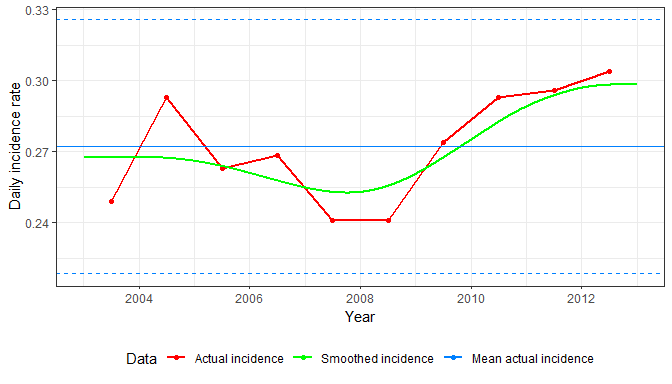Another useful diagnostic is to look at the age distribution of the incident cases.

ggplot(prevsim, aes(age, y=..count..)) +
geom_line(stat='density') +
xlim(0, 100) +
labs(x='Age (years)', y='Number incident cases') +
theme_bw()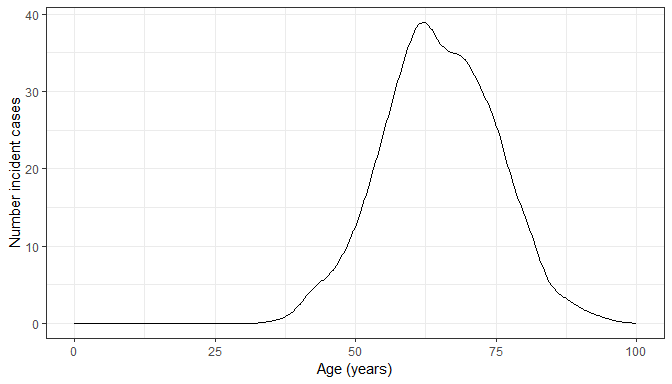# Survival modelling

In the default implementation, survival is modelled using a parametric fit with a specified distribution. It is highly recommended that the user inspects this model to assess its suitability. In this example, we’ll use the prevsim data set and test the assumptions of a Weibull model acting on the sex and age covariates, since these are common demographic factors that are likely to be used in prevalence estimation.

First, it is always useful to plot the Kaplan-Meier estimator of the data, both as a whole and stratified by age to visually inspect for any inconsistencies:

km <- survfit(Surv(time, status) ~ 1, data=prevsim)
plot(km, lwd=2, col="blue", main="Overall Survival", xlab="Days",
ylab="Survival probability")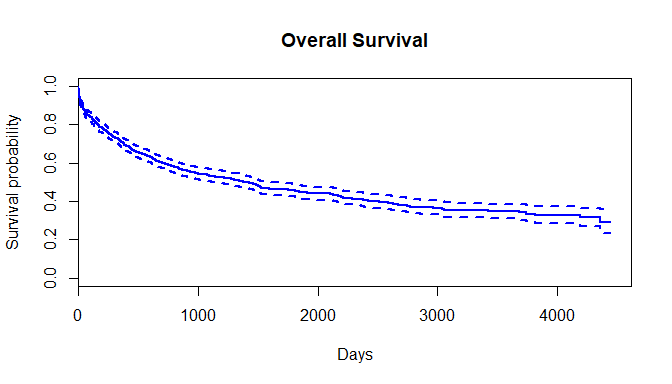ages = c(55, 65, 75, 85, 100)
km2 <- survfit(Surv(time, status) ~ cut(age, breaks=ages), data=prevsim)
plot(km2, lwd=2, col=1:length(ages), main="Survival stratified by age", xlab="Days",
ylab="Survival probability")
legend("topright", legend=substring(names(km2$strata), 25, 32), lty = 1, col=1:length(ages))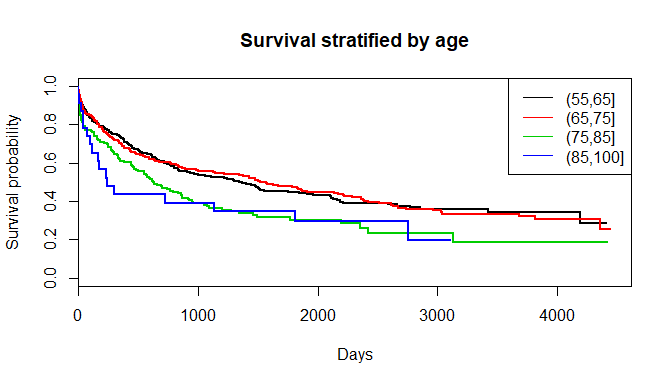It is also a useful diagnostic aid to plot the survival curve for each year of the registry to determine whether there is any inhomogeneity: plot(km, lwd=2, col="blue", mark.time=F, conf.int=T, xlab="Days", ylab="Survival probability") num_reg_years <- 9 registry_years <- sapply(0:9, function(x) as.Date(paste0(2004+x, "-01-30"))) sapply(seq(num_reg_years), function(i) lines(survfit(Surv(time, status) ~ 1, data=prevsim[prevsim$entrydate >=
registry_years[i] &
prevsim$entrydate < registry_years[i + 1], ]), mark.time = F, conf.int = F))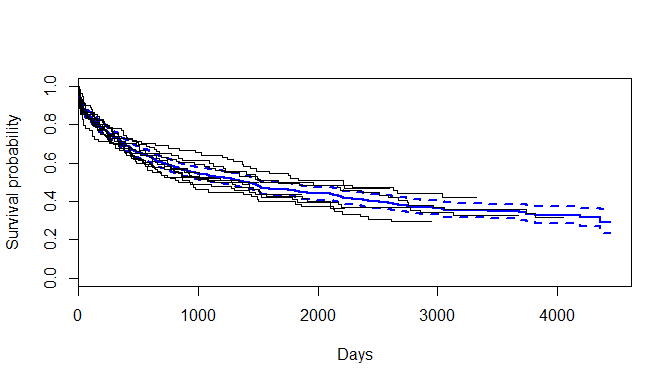The effect of age on hazard can be visualized to determine if there are any non-proportional effects, by inspecting Schoenfeld residuals from a Cox model. This is easily done using the cox.zph function from the survival package. cx <- coxph(Surv(time, status) ~ age, data=prevsim) cxp <- survfit(cx, newdata=data.frame(age=sapply(seq(length(ages) - 1), function(i) mean(c(ages[i], ages[i + 1]))))) plot(cox.zph(cx)) lines(cxp, lwd=2, col=1:length(ages), lty=2, mark.time=F)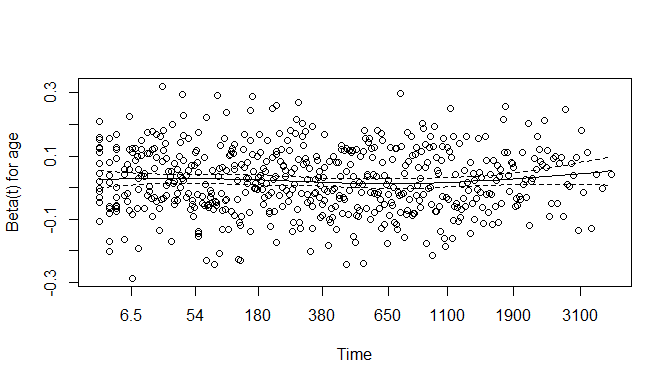An overall test of the proportional hazards assumption may be made. cox.zph(cx) ## rho chisq p ## age -0.0106 0.0647 0.799 ## Functional form of age The standard standard assumption in a survival model is that continuous variables have a linear relationship with log-hazard. When looking at the impact of age on disease survival, we highly recommend investigating whether a non-linear functional form provides a more appropriate fit. In particular, the rms package provides the cph function to fit a Cox model, to which restricted cubic splines can be placed on covariates using rcs as demonstrated below. Plotting log hazard as a function of age provides a visual means of assessing model fit, to be used alongside inspection of the model coefficients. library(rms) library(dplyr) mod_spline <- cph(Surv(time, status) ~ rcs(age, df=4), prevsim, x=TRUE, y=TRUE, surv=TRUE, time.inc=1) # Calculates log hazard linear predictor at 100 linearly separated ages between the limits # in the registr data age_range <- seq(min(prevsim$age), max(prevsim$age), length.out=100) preds <- predict(mod_spline, newdata=age_range, se.fit=T) preds_df <- as.data.frame(preds) %>% rename(lp=linear.predictors, se=se.fit) %>% mutate(age = age_range, upper = lp + 2 * se, lower = lp - 2 * se) ggplot(preds_df, aes(x=age, y=lp)) + geom_ribbon(aes(ymin=lower, ymax=upper), colour='#d2d2d2', alpha=0.30) + geom_line(colour='#0080ff', size=1.2) + theme_bw() + labs(x='Age', y='Log relative hazard')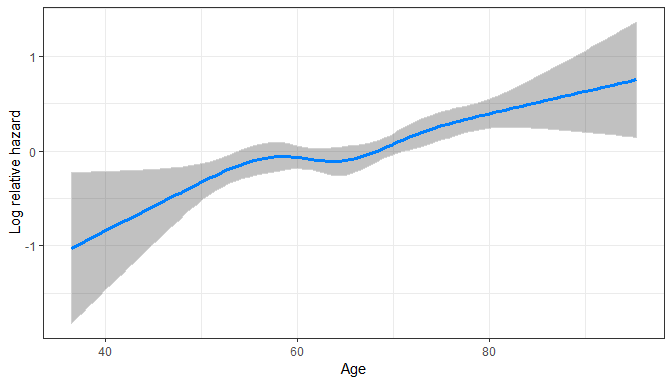For example, in this situation there isn’t enough evidence to suggest a non-linear effect provides a better model fit over a linear relationship. mod_spline ## Cox Proportional Hazards Model ## ## cph(formula = Surv(time, status) ~ rcs(age, df = 4), data = prevsim, ## x = TRUE, y = TRUE, surv = TRUE, time.inc = 1) ## ## Model Tests Discrimination ## Indexes ## Obs 1000 LR chi2 31.45 R2 0.031 ## Events 558 d.f. 4 Dxy 0.145 ## Center 2.8919 Pr(> chi2) 0.0000 g 0.259 ## Score chi2 32.52 gr 1.296 ## Pr(> chi2) 0.0000 ## ## Coef S.E. Wald Z Pr(>|Z|) ## age 0.0513 0.0246 2.09 0.0370 ## age' -0.1745 0.1128 -1.55 0.1221 ## age'' 0.9259 0.6024 1.54 0.1242 ## age''' -1.2260 0.8784 -1.40 0.1628 ##  # Prevalence estimates As a reminder, prevalence is estimated using incidence and survival data from n years. However, registry data (and thus known incidence and survival) data may only be known for r years, where r <= n. If r < n, the remaining n-r years of incidence and survival are simulated using Monte Carlo techniques. If the incidence and survival models are well specified then the prevalence estimates should be reliable, however, it is beneficial to check the performance of these bootstrapped models (their variance in particular) before drawing any conclusions from the results. prevalence_total <- prevalence(index='2013-01-30', num_years_to_estimate=c(3, 5, 10, 20), data=prevsim, inc_formula = entrydate ~ sex, surv_formula = Surv(time, status) ~ age + sex, dist='weibull', population_size = 1e6, death_column = 'eventdate') ## Comparison between simulated and counted prevalence As a test of whether the model is predicting reasonable values of prevalence, we can use the fact that we can directly measure the discrepancy between the predicted and actual prevalence for the available registry years. This difference can be formally tested with an exact Poisson test of the counted prevalence from both the simulated estimate and the known registry value; the resulting p-value resulting is returned as an attribute of a prevalence object, called pval. prevalence_total$pval
##  0.5087715

For this model, there is no evidence to reject the null hypothesis.

This can also be calculated manually with the test_prevalence_fit function.

test_prevalence_fit(prevalence_total)
##  0.5087715

## Diagnosing incidence models

We do not provide any functions for diagnosing the bootstrapped incidence models, however, all the objects are available in the inc_models attribute of the returned prevalence object and can be used to check for any errors in fitting. If the default homogeneous Poisson process model is being used then the techniques described earlier can be applied.

## Diagnosing survival models

To inspect the distribution of the bootstrapped survival models (see Crouch et al. 2014 for details), a survfit.prev object can be constructed using the usual survfit call, accepting a data frame of new data, defaulting to the average covariate values in the registry data. In the example below, survival probability is estimated for a 60 year old male:

prevsurv <- survfit(prevalence_total, newdata=data.frame(age=60, sex='M'))
prevsurv
## Survival probability calculated at 4444 timepoints, across 1000 bootstraps.

The summary method provides N-year survival probabilities, with N specified as an argument vector:

summary(prevsurv, years=c(1, 3, 5, 10))
## Survival probability estimated using 1000 bootstrap survival curves:
## 1 year survival: 0.716 (0.683 - 0.746)
## 3 year survival: 0.551 (0.508 - 0.59)
## 5 year survival: 0.459 (0.412 - 0.502)
## 10 year survival: 0.326 (0.277 - 0.372)

Plotting this object displays the survival curve of a Weibull model using the original data set (orange), along with a 95% confidence band derived using the bootstrapped models. This plot is useful to assess the variability of the survival models. Further manual inspection can be carried out by looking at the objects themselves, saved in the surv_models attribute of the prevalence object.

plot(prevsurv)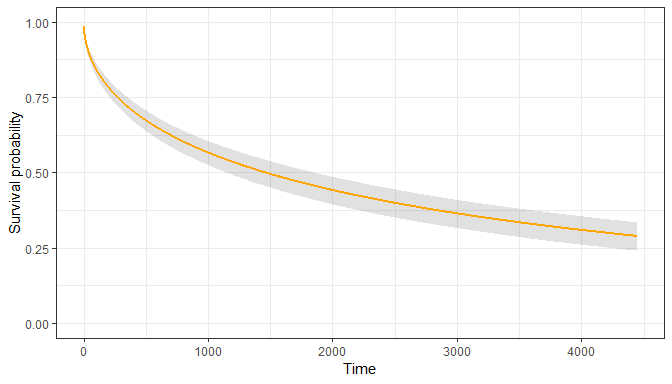## Simulated population

The simulated attribute of the prevalence object holds a data.table with the incident population from every simulation, along with derived fields indicating whether they contributed to prevalence at the index date of any years of interest. It can be used as an overall check of the appropriateness of the generated incidence population, as well as identifying any discrepancies in the survival modelling.

knitr::kable(head(prevalence_total\$simulated))
sim sex age incident_date alive_at_index prev_3yr prev_5yr prev_10yr prev_20yr prev_registry
1 M 71.96575 1993-02-03 0 0 0 0 0 0
1 M 49.98995 1993-02-03 1 0 0 0 1 0
1 M 75.26040 1993-02-16 0 0 0 0 0 0
1 M 75.29573 1993-03-10 0 0 0 0 0 0
1 M 61.02602 1993-03-22 0 0 0 0 0 0
1 M 70.35218 1993-03-27 1 0 0 0 1 0